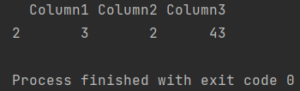Pandas How To Uncategorized How to print index

# How to print index

Here are tips on how to print index in Pandas.This is easy to print a column. But how to print a row in Pandas?

## how to print index with loc

To access a row using loc function you need to use a row index label as an argument.

```import pandas as pd

my_df = pd.DataFrame(
{'Column1': ['1', '4', '3', '4'],
'Column2': ['5', '6', '2', '2'],
'Column3': ['33', '10', '43', '12']},
index=['indexA', 'indexB', 'indexC', 'indexD'])

print(my_df.loc[['indexB']])

```

Output:

```       Column1 Column2 Column3
indexB       4       6      10

```

## how to print index with iloc

To access a row using loc function you need to use a row index position as an argument.

```import pandas as pd

my_df = pd.DataFrame(
{'Column1': ['1', '4', '3', '4'],
'Column2': ['5', '6', '2', '2'],
'Column3': ['33', '10', '43', '12']})

print(my_df.iloc[])
```

Output:

```  Column1 Column2 Column3
2       3       2      43
```

Outputs are the same.

Tags: ,

## How to add empty column in PandasHow to add empty column in Pandas

You can add an empty column to a Pandas DataFrame using the following code:

## How to select columns from list in PandasHow to select columns from list in Pandas

To select columns from a pandas dataframe, you can use the square bracket notation [] and pass the column names as a list inside it. Here’s an example:

## How to replace specific values in column in PandasHow to replace specific values in column in Pandas

To replace specific values in a column in Pandas, you can use the replace() method. This method allows you to replace one or more values with a new value or